Search by Topic

Resources tagged with Combinatorics similar to Tree Graphs:

Filter by: Content type:
Age range:
Challenge level:

There are 41 results

Broad Topics > Decision Mathematics and Combinatorics > CombinatoricsMagic W Wrap Up

Age 16 to 18 Challenge Level:

Prove that you cannot form a Magic W with a total of 12 or less or with a with a total of 18 or more.Russian Cubes

Age 14 to 16 Challenge Level:

I want some cubes painted with three blue faces and three red faces. How many different cubes can be painted like that?Transitivity

Age 16 to 18

Suppose A always beats B and B always beats C, then would you expect A to beat C? Not always! What seems obvious is not always true. Results always need to be proved in mathematics.Knight Defeated

Age 14 to 16 Challenge Level:

The knight's move on a chess board is 2 steps in one direction and one step in the other direction. Prove that a knight cannot visit every square on the board once and only (a tour) on a 2 by n board. . . .Ordered Sums

Age 14 to 16 Challenge Level:

Let a(n) be the number of ways of expressing the integer n as an ordered sum of 1's and 2's. Let b(n) be the number of ways of expressing n as an ordered sum of integers greater than 1. (i) Calculate. . . .Cube Net

Age 16 to 18 Challenge Level:

How many tours visit each vertex of a cube once and only once? How many return to the starting point?Postage

Age 14 to 16 Challenge Level:

The country Sixtania prints postage stamps with only three values 6 lucres, 10 lucres and 15 lucres (where the currency is in lucres).Which values cannot be made up with combinations of these postage. . . .Doodles

Age 14 to 16 Challenge Level:

Draw a 'doodle' - a closed intersecting curve drawn without taking pencil from paper. What can you prove about the intersections?Plum Tree

Age 14 to 18 Challenge Level:

Label this plum tree graph to make it totally magic!N000ughty Thoughts

Age 14 to 16 Challenge Level:

How many noughts are at the end of these giant numbers?Symmetric Tangles

Age 14 to 16

The tangles created by the twists and turns of the Conway rope trick are surprisingly symmetrical. Here's why!W Mates

Age 16 to 18 Challenge Level:

Show there are exactly 12 magic labellings of the Magic W using the numbers 1 to 9. Prove that for every labelling with a magic total T there is a corresponding labelling with a magic total 30-T.Magic W

Age 14 to 16 Challenge Level:

Find all the ways of placing the numbers 1 to 9 on a W shape, with 3 numbers on each leg, so that each set of 3 numbers has the same total.Magic Caterpillars

Age 14 to 18 Challenge Level:

Label the joints and legs of these graph theory caterpillars so that the vertex sums are all equal.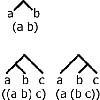Counting Binary Ops

Age 14 to 16 Challenge Level:

How many ways can the terms in an ordered list be combined by repeating a single binary operation. Show that for 4 terms there are 5 cases and find the number of cases for 5 terms and 6 terms.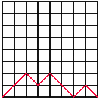Age 14 to 16 Challenge Level:

A walk is made up of diagonal steps from left to right, starting at the origin and ending on the x-axis. How many paths are there for 4 steps, for 6 steps, for 8 steps?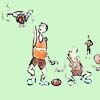Age 7 to 18 Challenge Level:

Libby Jared helped to set up NRICH and this is one of her favourite problems. It's a problem suitable for a wide age range and best tackled practically.Tangles

Age 11 to 16

A personal investigation of Conway's Rational Tangles. What were the interesting questions that needed to be asked, and where did they lead?Factorial Fun

Age 16 to 18 Challenge Level:

How many divisors does factorial n (n!) have?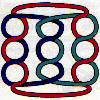Age 14 to 18

Some puzzles requiring no knowledge of knot theory, just a careful inspection of the patterns. A glimpse of the classification of knots, prime knots, crossing numbers and knot arithmetic.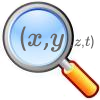Stage 5 Cipher Challenge

Age 16 to 18 Challenge Level:

Can you crack these very difficult challenge ciphers? How might you systematise the cracking of unknown ciphers?A Fine Thing?

Age 16 to 18 Challenge Level:

Second challenge cipherVital?

Age 16 to 18 Challenge Level:

Third challenge cipherUp a Semitone?

Age 16 to 18 Challenge Level:

Fifth challenge cipherIp?

Age 16 to 18 Challenge Level:

Seventh challenge cipherJluuis or Even Asutguus?

Age 16 to 18 Challenge Level:

Sixth challenge cipherLost in Space

Age 14 to 16 Challenge Level:

How many ways are there to count 1 - 2 - 3 in the array of triangular numbers? What happens with larger arrays? Can you predict for any size array?Deep Roots

Age 14 to 16 Challenge Level:

Find integer solutions to: $\sqrt{a+b\sqrt{x}} + \sqrt{c+d.\sqrt{x}}=1$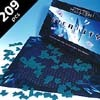The Eternity Puzzle

Age 16 to 18

A big prize was offered for solving The Eternity Puzzle, a jigsaw with no picture and every piece is the same on both sides. The finished result forms a regular dodecagon (12 sided polygon).Euler's Officers

Age 14 to 16 Challenge Level:

How many different ways can you arrange the officers in a square?Olympic Magic

Age 14 to 16 Challenge Level:

in how many ways can you place the numbers 1, 2, 3 … 9 in the nine regions of the Olympic Emblem (5 overlapping circles) so that the amount in each ring is the same?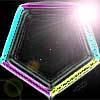Penta Colour

Age 14 to 16 Challenge Level:

In how many different ways can I colour the five edges of a pentagon red, blue and green so that no two adjacent edges are the same colour?Snowman

Age 14 to 16 Challenge Level:

All the words in the Snowman language consist of exactly seven letters formed from the letters {s, no, wm, an). How many words are there in the Snowman language?Semicircle

Age 16 to 18 Challenge Level:

Fourth challenge cipher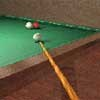Snooker Frames

Age 16 to 18 Challenge Level:

It is believed that weaker snooker players have a better chance of winning matches over eleven frames (i.e. first to win 6 frames) than they do over fifteen frames. Is this true?An Introduction to Computer Programming and Mathematics

Age 16 to 18

This article explains the concepts involved in scientific mathematical computing. It will be very useful and interesting to anyone interested in computer programming or mathematics.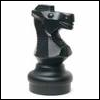Modular Knights

Age 16 to 18 Challenge Level:

Try to move the knight to visit each square once and return to the starting point on this unusual chessboard.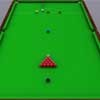Snooker

Age 16 to 18 Challenge Level:

A player has probability 0.4 of winning a single game. What is his probability of winning a 'best of 15 games' tournament?Molecular Sequencer

Age 14 to 18 Challenge Level:

Investigate the molecular masses in this sequence of molecules and deduce which molecule has been analysed in the mass spectrometer.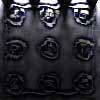Scratch Cards

Age 14 to 16 Challenge Level:

To win on a scratch card you have to uncover three numbers that add up to more than fifteen. What is the probability of winning a prize?In a Box

Age 14 to 16 Challenge Level:

Chris and Jo put two red and four blue ribbons in a box. They each pick a ribbon from the box without looking. Jo wins if the two ribbons are the same colour. Is the game fair?